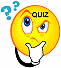Professor Freedman's
Elementary Algebra Homework Schedule
Spring 2023
(13 week course)

Text: Beginning Algebra by Miller, O'Neill & Hyde, 6th Edition, McGrawHill, Inc.,2022

For Tutorials on Varied Topics Written by Fellow Students go to: http://www.mathpower.com/tutorial.htm
 DateAssigned Assignments (Subject to Change) Week of 2/1/23 Homework Assigned for week of 2/1/23 Watch the following Videos on Signed Numbers & Algebraic Expressions Introduction to Algebra -Exponents, Absolute Values & Order of Operations Addition & Subtraction of Signed Numbers Multiplication & Division of Signed Numbers Order of Operations on Signed Numbers Evaluating an Algebraic Expression Commutative, Associative & Distributive Properties Combining Like Terms Go to aleks.com: Do Assignments # 1,2,3,4Go to aleks.com Quiz #1 in Aleks Homework Tips (Week 1) Week of 2/6/23 Homework Assigned for week of 2/6/23 Watch the following Videos: Solving Linear Equations (Part 1) Solving Equations (Identities & Contradictions) Solving Word Problems using Linear Equations Go to aleks.com: Do Assignments #5, 6 & 7Go to aleks.com Quiz #2 in Aleks Homework Tips (Week 2) Week of 2/13/23 Homework Assigned for week of 2/13/23 Watch the following Videos: Literal Equations Graphing Inequalities Solving Inequalities Identifying Solutions of 2 Variable Equations (Intro to Graphing) Plotting Points Graphing Straight Lines Slope of a Line Go to aleks.com Assignment #8 Literal Equations , Assignment 9 Inequalities, Assignment #10 Intro to Graphing, Assignment #10A (Graphing Straight Lines), Assignment #11 (Slope of a Line)Go to aleks.com Quiz #3 in Aleks Practice Test on Chapters 1,2,3 (Homework 11A) Week of 2/20/23 Homework Assigned for week of 2/20/23 Watch the following Videos: Solving Systems of Equations by Addition (Part 1) Solving Systems of Equations by Addition (Part 2) Inconsistent & Dependent Systems Assignment #12 (Solving Systems of Equations by Adding) AND Major Test on Chapters 1,2,3 and part of 4 (Including Systems of Equations) Week of 2/27/23 Watch the following Videos: Word Problems Using Systems of Equations Solving Systems of Equations by Substitution Watch the following Videos on Exponent Rules : Multiplying & Dividing Variables Zero Exponents Negative Exponents Writing a Polynomial in Standard Form Addition & Subtraction of Polynomials Go to aleks.com Assignment #13 (Solving Word Problems Using Systems of Equations & Solving Systems by Substitution)Assignment #14 (Exponent Rules) Assignment #15 (Addition & Subtraction of Polynomials)Go to aleks.com Quiz #4 on Systems of Equations and QUIZ #5 Exponent Rules & Polynomials Week of 3/6/23 Watch the following Videos: Multiplication of Polynomials Part 1 Multipliction of Polynomials FOIL Part 2 Polynomial Special Products Polynomial Division Assignment #16 (Multiplication of Polynomials) Assignment #17 (Division of Polynomials) Go to aleks.com STUDY FOR MIDTERM EXAM Homework Assignment #18 (Practice Midterm Exam) Week of 3/20/23 SPRING BREAK Week of 3/27/23 STUDY FOR MIDTERM EXAM - The MIDTERM EXAM covers everything in the Practice Test AND Polynomial Division from Assignment# 17. It does NOT include material from asssignments #19&20. Watch the following Videos: Factoring Trinomials with Leading Coefficient of One Go to aleks.com Assignment #19 Factoring Greatest Common Factor) & Assignment #20 ( Factoring Difference of Two Squares), Assignment #21 (Factoring Trinomials with a Leading Coefficient of One) MIDTERM EXAM DUE Week of 4/3/23 Watch the following Videos on Factoring : Go to aleks.com Assignment #22 ( Factoring Trinomials woith Leading Coeffficient other than One by Trial and Error) Assignment #23 (Solving Quadratic Equations by Factoring)Go to aleks.com Quiz #5 on Factoring Trinomials and Solving Quadratic Equations by Factoring Week of 4/10/23 Watch the following Videos:    Multiplying & Dividing Algebraic Fractions with Factoring Getting An Algebraic Common Denominator  Go to aleks.com Assignment #24 (Simplifying Algebraic Fractions) , Assignment #25 (Multiplying and Dividing Algebraic Fractions),Assignment #26 Getting LCD and Adding & Subtracting Algebraic Fractions with Same DenominatorsGo to aleks.com Quiz #6 on Simplifying, Multiplying and Dividing Algebraic Fractions, Adding & Subtracting with Same Denominator Week of 417/23 Watch the following Videos: Getting An Algebraic Common Denominator Addition & Subtraction of Rational Expressions with Different Denominators (Part 1) Addition & Subtraction of Rational Expressions with Different Denominators (Part 2) Solving Rational Equations (Equations with Algebraic Fractions) ,Assignment #27 Adding & Subtracting Algebraic Fractions with Different Denominators Assignment 28 (Solving Rational Equations) Assignment #29 (PRACTICE TEST on Factoring & Algebraic Fractions - STUDY GUIDE) Week of 4/24/23 Watch the following Videos: Simplifying Radicals (Numbers) Simplifying Radicals with Variables Go to aleks.com MAJOR TEST on FACTORING, ALGEBRAIC FRACTIONS AND Assignment #30 - PRACTICE FINAL EXAM - STUDY GUIDE Week of 5/1/23 Watch the following Videos: Go to aleks.com Final Exam FINAL EXAM